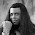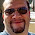## Thursday, January 7, 2016

### HP 15C: Digital Root, Modulus, and 2D Coordinate Rotation

HP 15C:  Digital Root, Modulus, and 2D Coordinate Rotation

Note:  steps are for reference points only.

HP 15C: Digital Root

Input: Enter an integer and execute Label A (or whatever label you want to assign).

Program:

 Step Key Key Code 001 LBL A 42, 21, 11 002 ENTER 36 003 ENTER 36 004 1 1 005 - 30 006 9 9 007 ÷ 10 008 INT 43, 44 009 9 9 010 * 20 011 - 30 012 RTN 43, 32

Formula used:  (Define DR(n) as the digital root function)
DR(n) = n – 9 * int((n-1)/9), n > 0

DR returns the sum of n’s digits and repeats until a single digit remains.

Examples:
DR(4514) = 5
DR(9376) = 7
DR(636088) = 4
DR(761997) = 3

HP 15C:  Modulus Function

Input:
Y:  A
X:  B

The program calculates A mod B.

Program:

 Step Key Key Code 001 LBL B 42, 21, 2 002 STO 2 44, 2 003 X<>Y 34 004 STO 1 44, 1 005 X<>Y 34 006 ÷ 10 007 FRAC 42, 44 008 RCL* 2 45, 20, 2 009 STO 3 44, 3 010 RCL 1 45, 1 011 RCL* 2 45, 20, 2 012 TEST 1 (x > 0) 43, 30, 1 013 GTO 1 22, 1 014 RCL 3 45, 3 015 RCL+ 2 45, 40, 2 016 STO 3 44, 3 017 RTN 43, 32 018 LBL 1 42, 21, 1 019 RCL 3 45, 3 020 RTN 43, 32

Formula Used:
A mod B = B * frac(A/B)
Add B to result if A*B < 0.

Registers Used:
R1 = A
R2 = B
R3 = A mod B

Examples:
A = 48, B = 3, result = 0
A = 41.3, B = 12, result = 5.3
A = 48, B = -7, result = -1
A = -50.2, B = 36, result = 21.8

HP 15C:  2D Coordinate Rotation

Input: Store the following:  X in R4, Y in R5, and θ in R3.  Run the program.

Results are stored in R6 and R7, for X’ and Y’, respectively. X’ is displayed first, press R/S to get Y’.

This program uses the Polar to Rectangular conversion.

Program:

 Step Key Key Code 001 LBL C 42, 21, 13 002 RCL 3 45, 3 003 RCL 4 45, 4 004 >R 42, 1 005 STO 6 44, 6 006 X<>Y 34 007 STO 7 44, 7 008 RCL 3 45, 3 009 RCL 5 45, 5 010 >R 42, 1 011 RCL 7 45, 7 012 + 40 013 STO 7 44, 7 014 X<>Y 34 015 CHS 16 016 RCL 6 45, 6 017 + 40 018 STO 6 44, 6 019 R/S 31 020 X<>Y 34 021 RTN 43, 32

Formulas Used:
X’ = X * cos θ – Y * sin θ
Y’ = X * sin θ + Y * cos θ

Examples:
X (R4) = 1, Y (R5) = 2, θ (R3) = 30°.   Results: X’ (R6) ≈ -0.1340,  Y’ (R7) ≈ 2.2321
X (R4) = 6.45, Y (R5) = 5.25, θ (R3) = 176°.  Results:  X’ (R6) ≈ -6.8005, Y’ (R7) ≈ -4.7872

Until next time, be safe everyone!

Eddie

This blog is property of Edward Shore.  2016

1.Thank you. I needed a modulus program for my HP 11 C.

1.Glad I can help!

Eddie

2.And thank you, Cowzilla.

### Retro Review: Radio Shack EC-2024

Retro Review:  Radio Shack EC-2024 Quick Facts Company:  Radio Shack Years in Production: Around 1994 Original Price: \$24.99 Bat...